# 理解CSS3 transform中的Matrix(矩阵)

2015-01-26 15:54:00
zengde

http://www.zhangxinxu.com/wordpress/?p=2427

## 理解CSS3 transform中的Matrix(矩阵)

### 一、哥，我被你吓住了

CSS同行间：

CSS门槛低，无需程序基础或数学逻辑能力，也能做出点自我感觉不错的东西。然而，你自己也应该清楚的，一般你能轻松学到的东西，别人也可以。因此，如果你想挤进那20%的行列，就要学到一般人学不到的深度，学到一般人学不了的东西。自然，是需要更多额外的努力的。如果每次你都比别人努力一点点，何愁不比他人高出几等。人年轻的时候，贵在坚持！

### 二、何为矩阵？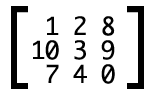CSS3中的矩阵
CSS3中的矩阵指的是一个方法，书写为matrix()和matrix3d()，前者是元素2D平面的移动变换(transform)，后者则是3D变换。2D变换矩阵为3*3, 如上面矩阵示意图；3D变换则是4*4的矩阵。

```.trans_skew { transform: skew(35deg); }
.trans_scale { transform:scale(1, 0.5); }
.trans_rotate { transform:rotate(45deg); }
.trans_translate { transform:translate(10px, 20px); }
```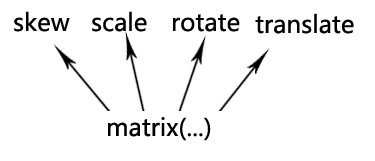OK，现在上面提到的CSS3矩阵解释应该说得通了。

### 四、transform与坐标系统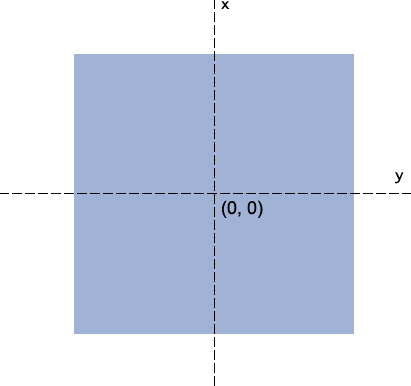`-webkit-transform-origin: bottom left;``transform-origin: 50px 70px;`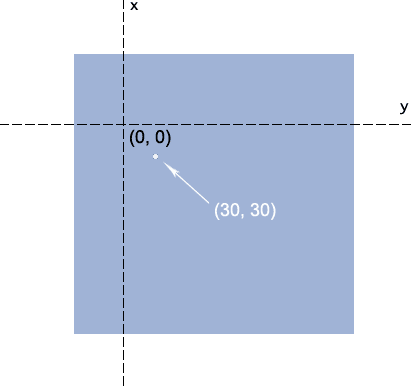### 五、准备好了没？重头戏来了

CSS3 transform的matrix()方法写法如下：

`transform: matrix(a,b,c,d,e,f);`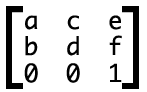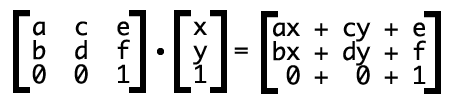//zxx:大学时候线性代数知识，懂的人这里可以直接跳过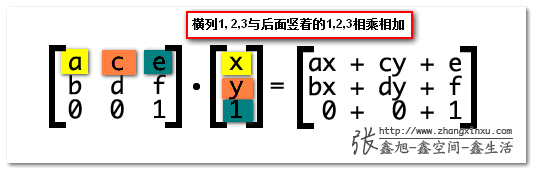`transform: matrix(1, 0, 0, 1, 30, 30); /* a=1, b=0, c=0, d=1, e=30, f=30 */`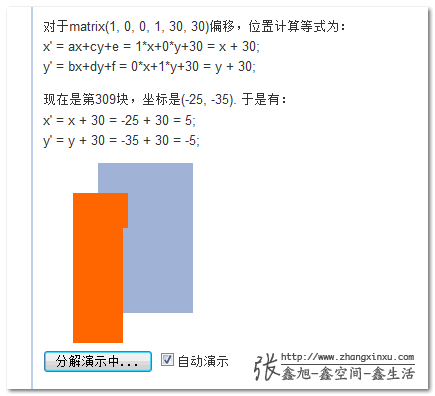//zxx:由于默认100毫秒间隔不断渲染，因此如果你电脑CPU或是浏览器hold不住，可以取消“自动演示”的勾选，然后，点击左边的按钮手动分步查看。

`transform: matrix(与我无关, 哪位, 怎么不去高考, 打麻将去吧, 水平偏移距离, 垂直偏移距离);`

### 六、transform matrix矩阵与缩放，旋转以及拉伸

`transform: matrix(1, 0, 0, 1, 30, 30);`

x' = ax+cy+e = s*x+0*y+0 = s*x;
y' = bx+dy+f = 0*x+s*y+0 = s*y;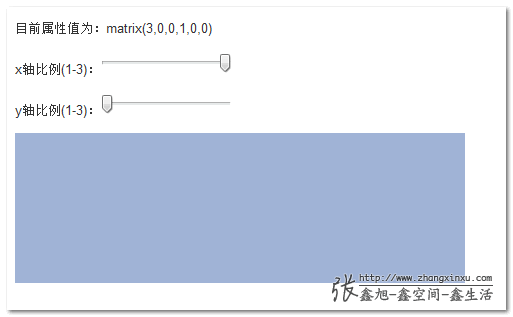`matrix(cosθ,sinθ,-sinθ,cosθ,0,0)`

```x' = x*cosθ-y*sinθ+0 = x*cosθ-y*sinθ
y' = x*sinθ+y*cosθ+0 = x*sinθ+y*cosθ```

`filter:progid:DXImageTransform.Microsoft.Matrix(M11=cosθ,M21=sinθ,M12=-sinθ,M22=cosθ');`

CS-SC：初三-上床，对称结构，这下忘不了了吧~~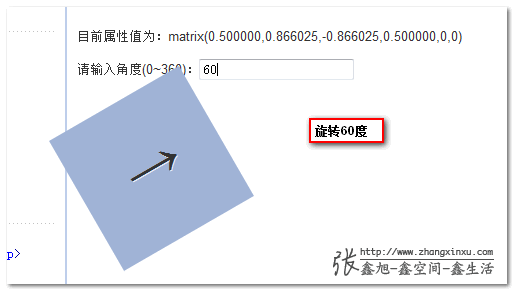`transform:rotate(30deg);`

`transform: matrix(0.866025,0.500000,-0.500000,0.866025,0,0);`

`matrix(1,tan(θy),tan(θx),1,0,0)`

```x' = x+y*tan(θx)+0 = x+y*tan(θx)
y' = x*tan(θy)+y+0 = x*tan(θy)+y```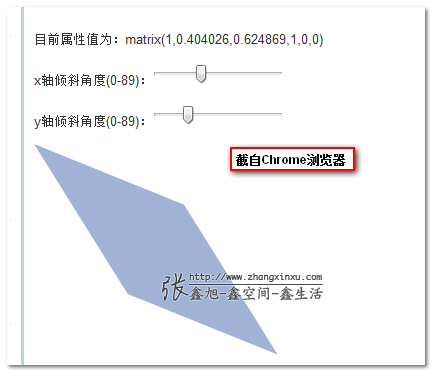### 七、既然有简单的skew, rotate..，那matrix有何用？

OK，这里就演示下，如何使用CSS3 transform matrix矩阵实现镜像效果。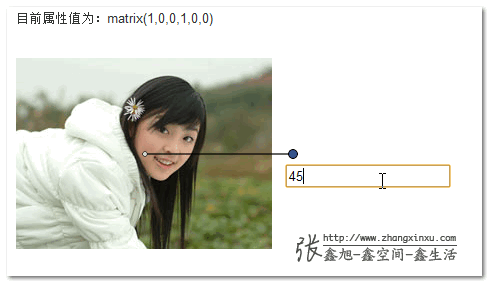demo页面中的一个轴是为了便于理解我加上的效果，实际上，在镜像对称的时候轴是看不见的。

`matrix((1-k*k) / (1+k*k), 2k / (1 + k*k), 2k / (1 + k*k), (k*k - 1) / (1+k*k), 0, 0)`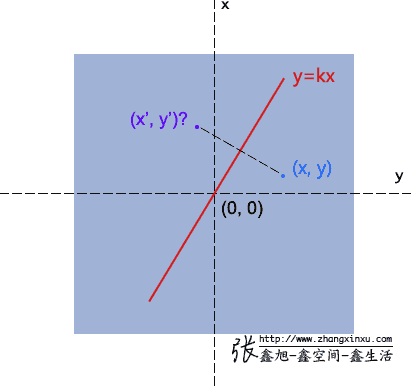(y-y') / (x - x') = -1/ k → ky-ky' = -x+x'
(x + x') / 2 * k = y + y' → kx+kx' = y+y'

x' = (1-k*k)/(k*k+1) *x + 2k/(k*k+1) *y;
y' = 2k/(k*k+1) *x + (k*k-1)/(k*k+1) *y;

x' = ax+cy+e;
y' = bx+dy+f;

a = (1-k*k)/(k*k+1);
b = 2k/(k*k+1);
c = 2k/(k*k+1);
d = (k*k-1)/(k*k+1);### 八、3D变换中的矩阵

3D变换虽然只比2D多了一个D，但是复杂程度不只多了一个。从二维到三维，是从4到9；而在矩阵里头是从3*3变成4*4, 9到16了。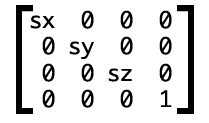`transform: matrix3d(sx, 0, 0, 0, 0, sy, 0, 0, 0, 0, sz, 0, 0, 0, 0, 1)`

（本篇完）